4WX Internet Search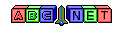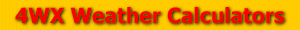# Mixing Ratio

From the user, an air temperature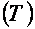, a dewpoint temperature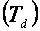, and a station pressure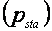are given.

The calculation of the mixing ratio is quite involved. In order to calculate the mixing ratio, a saturated vapor pressure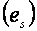must be computed for values of air temperature, and an actual vapor pressure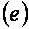must be computed for values of dewpoint temperature. But before the vapor pressures can be computed, the air temperature and/or dewpoint temperature must be converted to degrees Celsius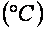. To see how to convert temperatures, see the link below:

Then, using the values of air temperature and/or dewpoint temperature the vapor pressure(s) can be computed. To see how to calculate the vapor pressure(s), see the link below:

vaporPressure.php

Next, the station pressuremust be converted to millibars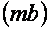or hectoPascal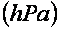To see how to convert the station pressure, see the link below:

Finally, the actual mixing ratioand/or saturated mixing ratio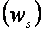can be calculated using the formula belowFor a bonus answer, the relative humidity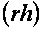can be calculated using the answers from the actual mixing ratio and the

saturated mixing ratio by using the formula below:Back to 4WX Weather Calculators index page

4WX Calculators Credit and disclaimer